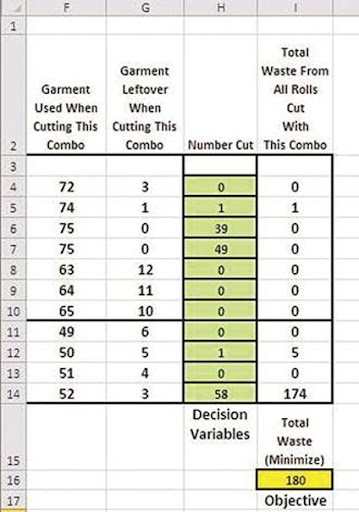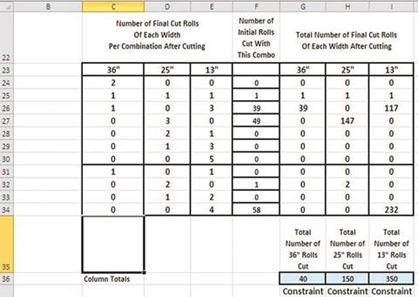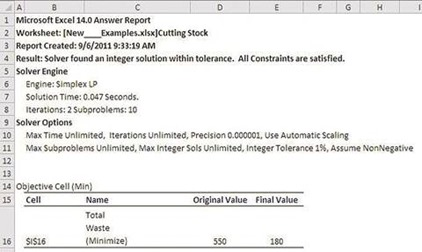# Optimizing the Cutting of Strips of Sheet to Minimize Waste With Excel Solver

This is another classic Solver problem with many possible variations. Cutting Stock problems involve cutting large sheets into the optimal number of smaller strips to meet customer orders while minimizing waste. The sheets can represent any type of material that come in a strip that is cut into smaller strips, such as a roll of steel. This problem involves rolls of garment that are cut to smaller sizes to meet individual customer orders.

## The Cutting Stock Problem

A garment factory produces rolls of garment in the following 2 sizes: rolls that are 75 inches wide and rolls that are 55 inches wide. Customers order garment rolls in the following 3 sizes: rolls that are 36 inches wide, rolls that are 25 inches wide, and rolls that are 13 inches wide.

The factory must cut the 75” rolls and 55” rolls in the correct number of 36”, 25”, and 13” rolls to meet individual customer orders while minimizing waste. Waste represents garment that is cut from the original 75” and 55” rolls which is not included in a customer order and is left-over.

## Excel Solver Problem Solving Steps

### Excel Solver Step 1 – Determine the Objective

In this case, the objective is to minimize the total waste left-over when satisfying each customer order. As seen in Step 3, Cutting Stock problems require listing all of the possible combinations that the original sheets can be cut in order to satisfy the customer’s order.

The amount of waste (leftover material) from each combination is shown. The total amount of waste of all combinations that are cut is also listed. The objective is to determine the correct number of each combination to cut that will meet the customer’s order and minimize the total amount of leftover, wasted garment. The Objective is to minimize the total amount of wasted garment. The cell is which the total waste from each order is calculated is the Objective Cell.

### Excel Solver Step 2 – Determine the Decision Variables

We are trying to determine how many of each combination to cut that will satisfy the customer’s order requirements and also minimize leftover wasted garment. The Decision Variables are the numbers of each type of cutting combination to cut.

### Excel Solver Step 3 – Build the Excel Equations That Combine the Objective With All Decision Variables

The yellow Objective Cell, I16, displays the total amount of leftover garment that will be wasted when a specific group of cutting combinations is applied to meet a customer order. This will be minimized.

The green Decision Variable cells, cells H4 to H14, display the number of each type of combination that will be cut to meet an individual customer order. Following is a complete view of the Excel model leading to the Objective.(Click On Image To See a Larger Version)

The following 2 images present an expanded view for better clarity of the left and right halves of the preceding complete Excel model. The 1st image is the left side of the model and list all possible cutting combinations and the waste left-over from each. The 2nd image shown is the right side of the model with the green Decision Variables and the yellow Objective Cell:(Click On Image To See a Larger Version)(Click On Image To See a Larger Version)

### Excel Solver Step 4 – List all Constraints(Click On Image To See a Larger Version)

The light blue Constraint cells in the model the preceding image link to the light blue user-controlled inputs outside of the model in the following image. The user-controlled inputs represent the customer’s order as follows:(Click On Image To See a Larger Version)

### Excel Solver Step 5 – Test the Excel Spreadsheet

Test the Excel spreadsheet completely before adding information to the Solver dialogue box. Make sure that any changes to Decision Variables produce the correct results in the Objective cell.

### Excel Solver Step 6 – Insert All Data into the Solver Dialogue Box

Input the Objective cell, Decision Variable cell, and all Constraints into the Solver dialogue box as follows:(Click On Image To See a Larger Version)

Note that the Decision Variables are Constrained to be integers because only whole numbers of garment rolls can be cut. The equality Constraints are the links between the number of garment rolls in the customer’s order and the model. Unconstrained variables are made nonnegative to ensure that there can be no negative numbers of rolls cut.

There are still a few more Constraints not shown in the preceding image that are shown as follows by scrolling down the slider bar next to the Constraints:(Click On Image To See a Larger Version)

All equations on the Excel spreadsheet are linear (1st order) so we can use the Simplex LP (Linear Programming) Solver engine for this optimization problem.

Step 3 shows the completed problem with Decision Variables that have been optimized by the Solver to maximize the Objective while staying within the problem’s Constraints.

Part 1

Note:

- The Solver Result

- How long Solver took to solve the problem

- The Solver Engine that was used and the Solver Options settings

- Where the Objective Cell was labeled in the Excel model for its name to appear as it does in Part 1 of the Answer Report(Click On Image To See a Larger Version)

Part 2

- Note that the Variable Cells contain the Decision Variables

- Note where the labels for each Decision Variable are placed in the Excel model so that the Decision Variable’s name will appear here in Part 2 of the Answer Report as it does

- Note the type of variable - Either Continuous or Integer (Integer, Binary, or Alldifferent)

- Note the Before and After values of each Decision Variable(Click On Image To See a Larger Version)

Part 3

- Note how each Constraint is labeled in the Excel model in order for the Constraint’s name to appear here in Part 3 of the Answer Report as it does

- Note which Constraints are binding (had their limits hit) and which aren’t.

- Note how much slack is still available in any Constraint that has not had its limit hit.

- Note any Integer Constraints (Integer, Binary, Alldifferent)(Click On Image To See a Larger Version)

Excel Master Series Blog Directory

Statistical Topics and Articles In Each Topic

• Histograms in Excel
• Bar Chart in Excel
• Combinations & Permutations in Excel
• Normal Distribution in Excel
• t-Distribution in Excel
• Binomial Distribution in Excel
• z-Tests in Excel
• t-Tests in Excel
• Hypothesis Tests of Proportion in Excel
• Chi-Square Independence Tests in Excel
• Chi-Square Goodness-Of-Fit Tests in Excel
• F Tests in Excel
• Correlation in Excel
• Pearson Correlation in Excel
• Spearman Correlation in Excel
• Confidence Intervals in Excel
• Simple Linear Regression in Excel
• Multiple Linear Regression in Excel
• Logistic Regression in Excel
• Single-Factor ANOVA in Excel
• Two-Factor ANOVA With Replication in Excel
• Two-Factor ANOVA Without Replication in Excel
• Randomized Block Design ANOVA in Excel
• Repeated-Measures ANOVA in Excel
• ANCOVA in Excel
• Normality Testing in Excel
• Nonparametric Testing in Excel
• Post Hoc Testing in Excel
• Creating Interactive Graphs of Statistical Distributions in Excel
• Solving Problems With Other Distributions in Excel
• Optimization With Excel Solver
• Chi-Square Population Variance Test in Excel
• Analyzing Data With Pivot Tables and Pivot Charts
• SEO Functions in Excel
• Time Series Analysis in Excel
• VLOOKUP
• Simplifying Useful Excel Functions

1.Wonderful article. Thanks for sharing such a useful post.

non woven fabric bag making machine

2.Hi, can you please share the excel sheet of this solver?

3.How are the values in H4 to H14 (green cells) determined? Is it a formula or an algorithm or just guessing?

4.Excellent .. Amazing .. I’ll bookmark your blog and take the feeds also…I’m happy to find so many useful info here in the post, we need work out more techniques in this regard, thanks for sharing.  JUNK HAULING